# 10张图，搞懂索引为什么会失效？

0收藏

### MySQL数据是如何存储的？

#### 聚集索引

``````CREATE TABLE `student` (
`id` int(11) NOT NULL AUTO_INCREMENT COMMENT '学号',
`name` varchar(10) NOT NULL COMMENT '学生姓名',
`age` int(11) NOT NULL COMMENT '学生年龄',
PRIMARY KEY (`id`),
KEY `idx_name` (`name`)
) ENGINE=InnoDB;``````

``````insert into student (`name`, `age`) value('a', 10);
insert into student (`name`, `age`) value('c', 12);
insert into student (`name`, `age`) value('b', 9);
insert into student (`name`, `age`) value('d', 15);
insert into student (`name`, `age`) value('h', 17);
insert into student (`name`, `age`) value('l', 13);
insert into student (`name`, `age`) value('k', 12);
insert into student (`name`, `age`) value('x', 9);``````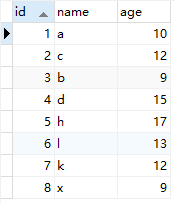mysql是按照页来存储数据的，每个页的大小为16k。

``show global status like 'innodb_page_size'``

1. 首先判断表中是否有非空的唯一索引，如果有，则该列即为主键。如果有多个非空唯一索引时，InnoDB存储引擎将选择建表时第一个定义的非空唯一索引作为主键
2. 如果不符合上述条件，InnoDB存储引擎自动创建一个6字节大小的指针作为索引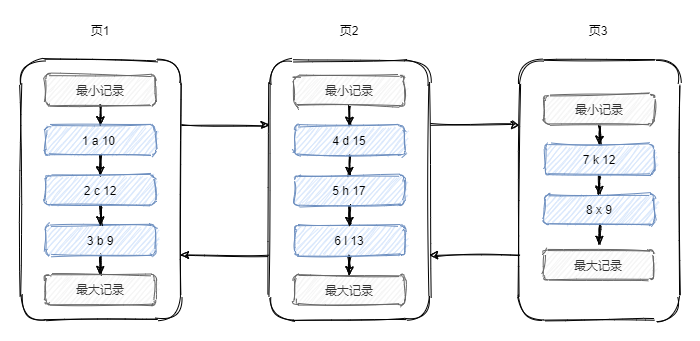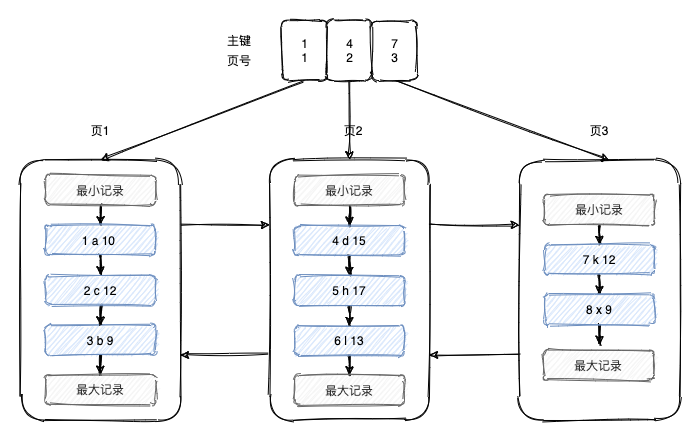1. 随着数据的增多，目录所需要的连续空间越来越大，并不现实
2. 当有一个页的数据全被删除了，则相应的目录项也要删除，它后面的目录项都要向前移动，成本太高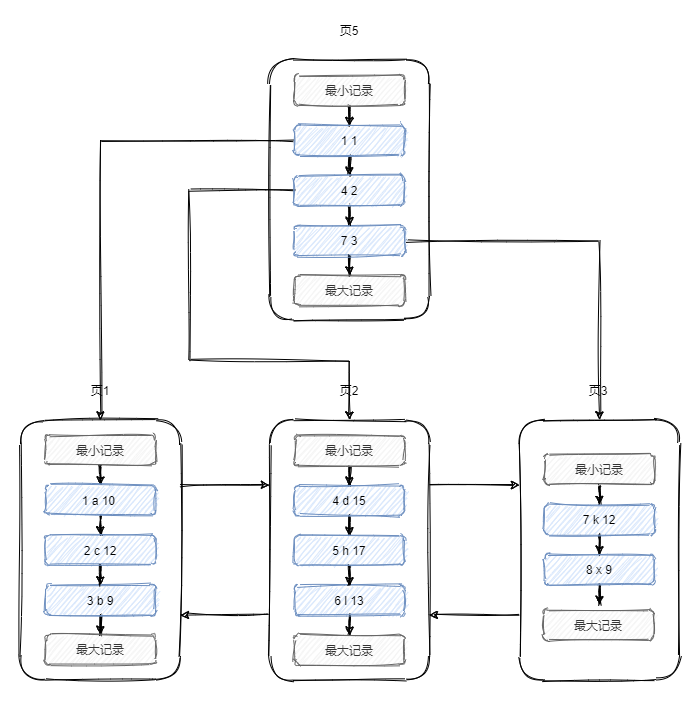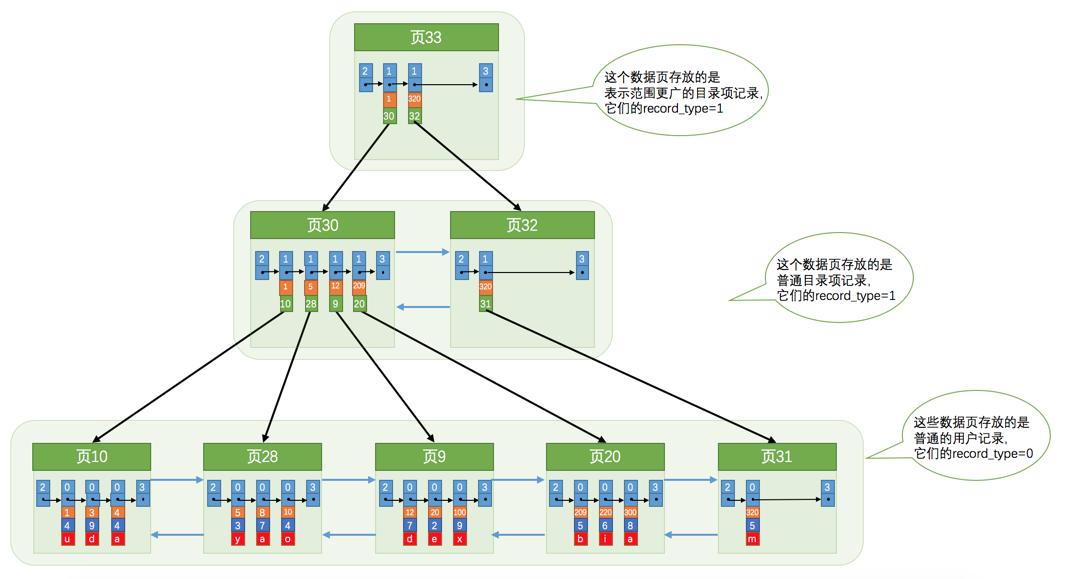#### 非聚集索引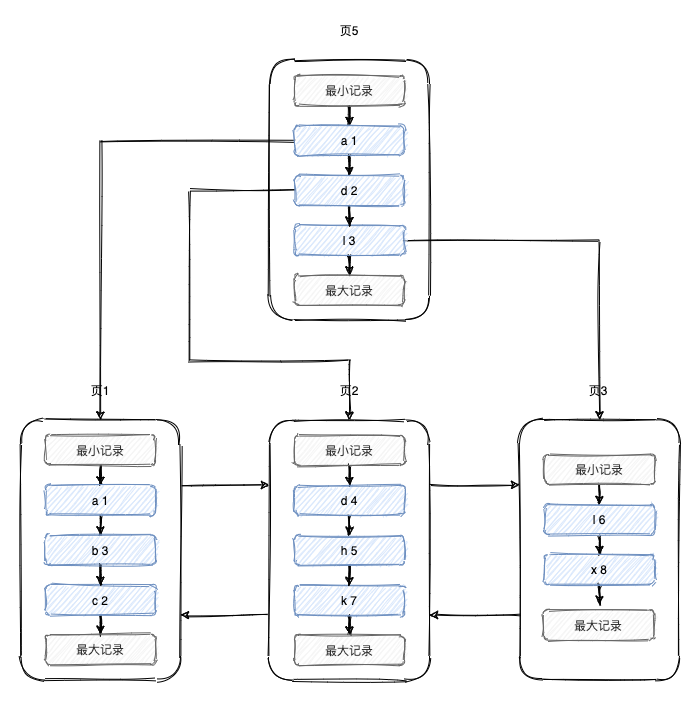#### 联合索引/索引覆盖

``````CREATE TABLE `teacher` (
`id` int(11) NOT NULL AUTO_INCREMENT COMMENT '教师编号',
`name` varchar(10) NOT NULL COMMENT '教师姓名',
`age` int(11) NOT NULL COMMENT '教师年龄',
`ismale` tinyint(3) NOT NULL COMMENT '是否男性',
PRIMARY KEY (`id`),
KEY `idx_name_age` (`name`, `age`)
) ENGINE=InnoDB;``````

``````insert into teacher (`name`, `age`, `ismale`) value('aa', 10, 1);
insert into teacher (`name`, `age`, `ismale`) value('dd', 12, 0);
insert into teacher (`name`, `age`, `ismale`) value('cb', 9, 1);
insert into teacher (`name`, `age`, `ismale`) value('cb', 15, 1);
insert into teacher (`name`, `age`, `ismale`) value('bc', 17, 0);
insert into teacher (`name`, `age`, `ismale`) value('bb', 15, 1);
insert into teacher (`name`, `age`, `ismale`) value('dd', 15, 1);
insert into teacher (`name`, `age`, `ismale`) value('dd', 12, 0);``````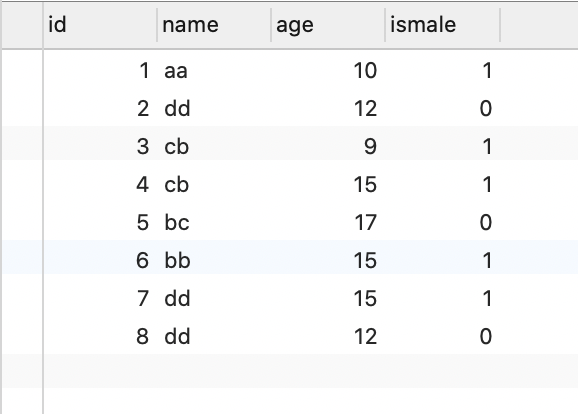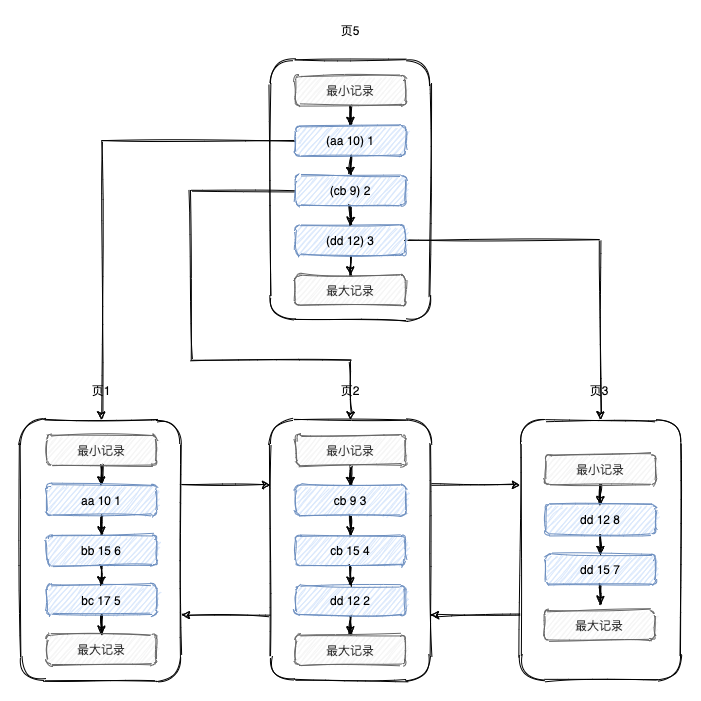``select * from student where name = 'aa';``

``select name, age from student where name = 'aa';``

#### 索引下推

``select * from student where name like '张%' and age = 10 and ismale = 1;``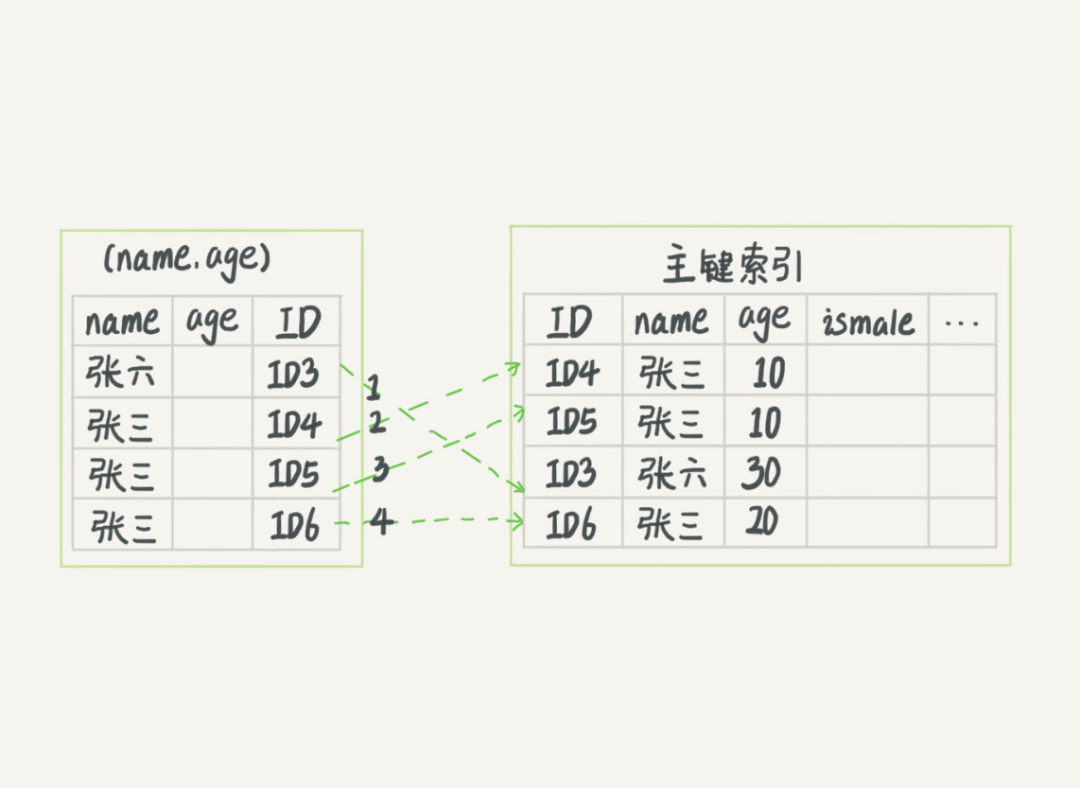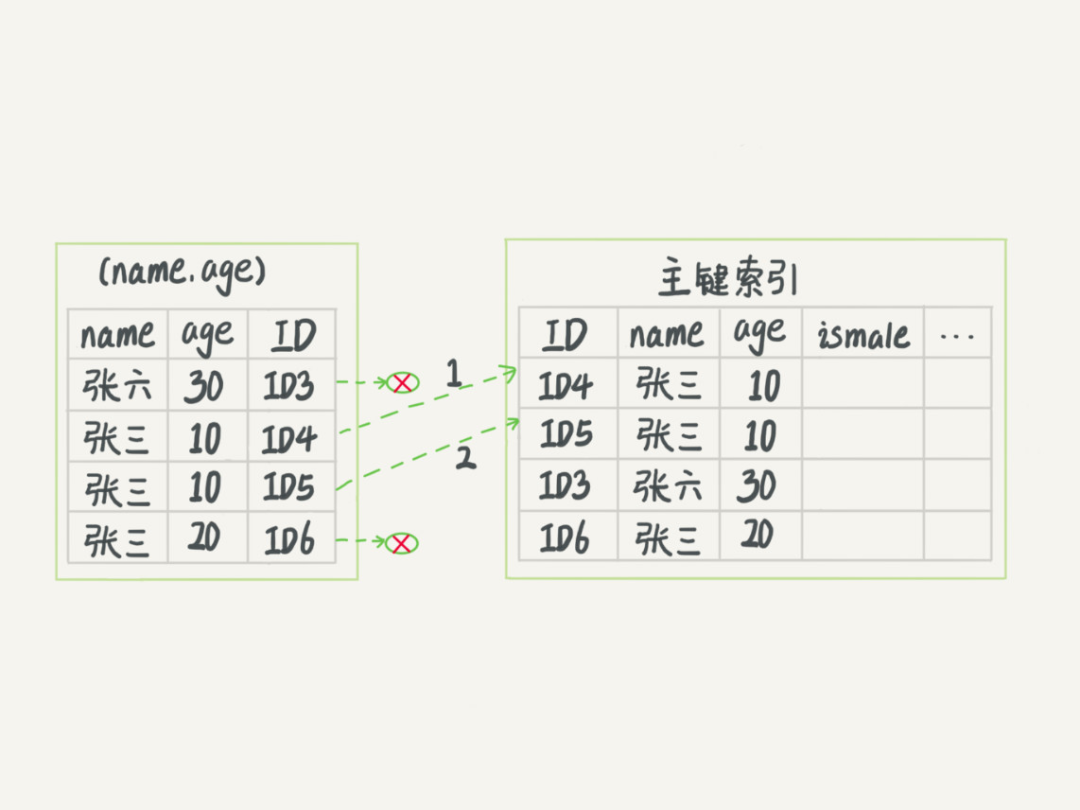### 最左前缀原则

#### 加速查询

1. 需要查询的列和组合索引的列顺序一致
2. 查询不要跨列

``````CREATE TABLE `people` (
`name` varchar(50) NOT NULL,
`country` varchar(50) NOT NULL,
) ENGINE=InnoDB DEFAULT CHARSET=utf8;``````

``````explain select * from people where name = "jack"
and address = "beijing" and country = "china"``````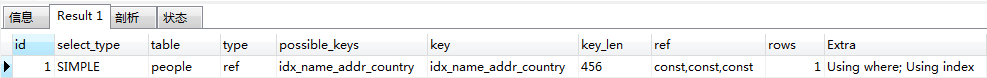type为ref，key_len为456=（50*3+2）*3，联合索引的所有列都使用了

``explain select * from people where name = "jack"``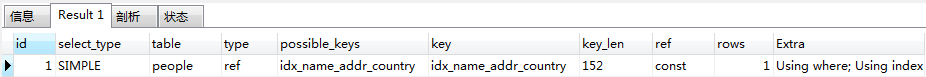type为ref，key_len为152=50*3+2，联合索引只使用了name列

``explain select * from people where address = "beijing"``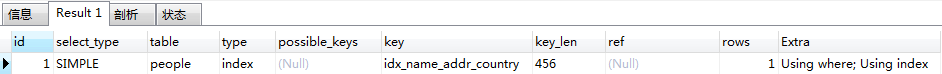type为index，表明查询的时候对整个索引进行了扫描，并没有加速查找。

 sql 是否使用索引 where a = x and b = x and c = x 是 where a = x and b = x 是，部分索引 where a = x 是，部分索引 where b = x 否，不包含最左列name where b = x and c = x 否，不包含最左列name

#### 加速排序

1. 通过有序索引顺序扫描直接返回有序数据
2. Filesort排序，对返回的数据进行排序

``explain select * from people order by name``Extra列只有Using index，即根据索引顺序进行扫描

``explain select * from people order by address``Extra列有Using filesort

order by 能使用索引排序

``````order by a
order by a,b
order by a,b,c
order by a desc, b desc, c desc
where a = const order by b,c
where a = const and b = const order by c
where a = const and b > const order by b,c``````

order by 不能使用索引进行排序

``````order by b
order by c
order by b, c
order by a asc, b desc, c desc //排序不一致
where g = const order by b,c //丢失a索引
where a = const order by c //丢失b索引
where a = const order by a,d //d不是索引的一部分
where a in (...) order by b,c //范围查询``````

### 索引为什么会失效？

1. 不要在索引列上进行运算或使用函数
2. 前导模糊查询不会使用索引，例如 like %李
3. 负向条件索引不会使用索引，建议用in。负向条件有：!=、<>、not in、not exists、not like 等帖子
视频
声望
粉丝
最近发布
热门推荐
社区精华内容